# Mathematics - Signals and Systems - Exercises on the Laplace Transform

in StemSocial5 months ago[Image1]

## Introduction

Hey it's a me again @drifter1!

Today we continue with my mathematics series about Signals and Systems in to get into Exercises on the Laplace Transform.

So, without further ado, let's dive straight into it!

## Small Refresher

The Laplace Transform is a generalization of the Fourier Transform for a complex number exponent: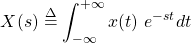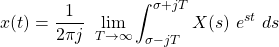Using it sometimes simplifies calculations for LTI systems, but can also allow analysis of systems that don't converge using the more-specific Fourier Transform. The Laplace Transform has similar properties to the Fourier Transform, which include linearity, time- and frequency-shifting, complex conjugation, multiplication-convolution, differentatition-integration etc. The main difference is that an region-of-convergence (ROC) needs to be specified in addition to the algebraic expression of a system. The ROC can sometimes be found easily through common system properties such as causality and stability, which are only satisfied by some ROCs.

## Laplace Transform and ROC Examples [Based on 20.9 from Ref1]

Let's determine the Laplace transform, pole-zero locations and associated ROC for each of the following cases: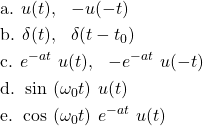### a.

The signal u(t) is one of the elementary signals, which is (like all cases throughout this example) included in Laplace Transform pair tables.

Both of these signals have the same algebraic Laplace Transformm, which is: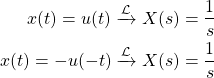and both signals also have the same pole (root of denominator) at the center of the axes (point O).

Their difference is only noticable in the ROC, where u(t) takes the right-side s-plane, whilst -u(-t) takes the left-side s-plane, as shown below.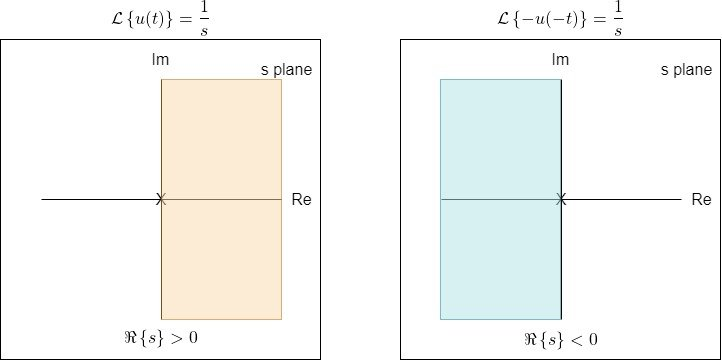### b.

The unit impulse function δ(t) and the time-shifted δ(t - t0) have an ROC which includes the complete s plane. Of course, the impulse function has an Laplace Transform of 1, similar to the Fourier Transform. And so, by simply applying the time-shifting property its easy to find out the Laplace Transform of the time-shifted δ(t - t0).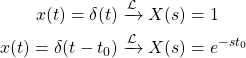### c.

The exponential functions are also transform table pair cases, with the same algebraic Laplace transform, if a is left as is: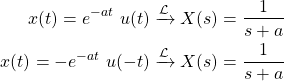Both have a pole at the point -a on the Real-axis, and one has an ROC to the right-side of that pole, whilst the other has an ROC to the left of it, as shown below.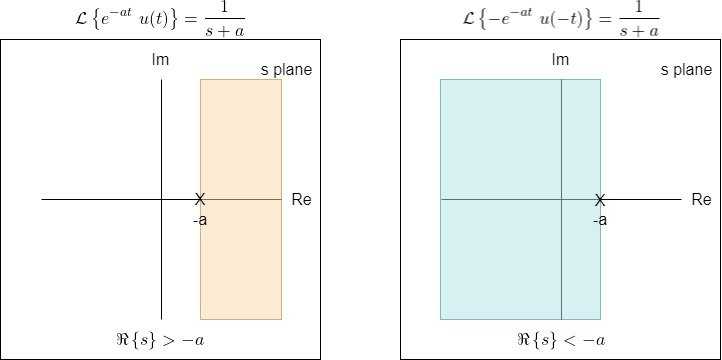### d.

The sinusoidal signals are also known cases, which are quite interesting on the s-plane. More specifically, the Laplace Transform is given by: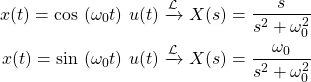In the case of the sine function, their are two roots for the denominator, which means that their are two poles, one at ω0 and one at 0 of the Imaginary-Axis. As such, the ROC is basically the right-side of the s-plane.

This leads to the pole-zero diagram shown below.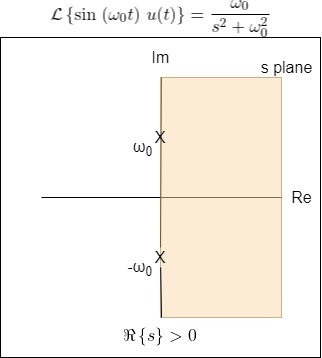### e.

Multiplying the sinuosidal signals of the previous example by an exponential, which as we know is frequency-shifting, leads to an ROC which is more closely linked to the ROC of such an exponential. In the case of the sine signal, again only poles can be found, and that's why this example is about the cosine signal, which includes a zero (root at the nominator).

Either way, by simply applying the frequency-shifting property, the Laplace Transform of such sinuosidal signals looks as follows: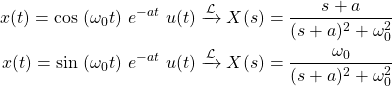The pole-zero diagram and ROC is shown below.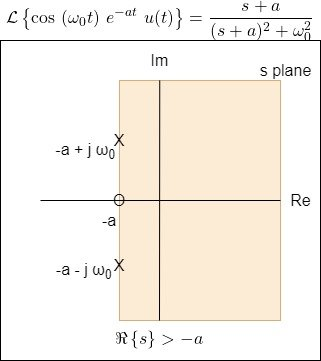## LTI System Analysis Example [Based on 21.4 from Ref1]

Consider a continuous-time LTI system, for which the input x(t) and output y(t) are related by the following LCCDE: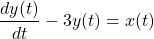Let's:

1. determine the Laplace transform of the impulse response h(t), H(s) and sketch the pole-zero diagram
2. specify the ROC for the following cases:
1. The system is causal
2. The system is anti-causal
3. The system is stable
3. determine h(t) if the system is causal

### 1.

Taking the Laplace Transform of the two sides of the differential equations yields: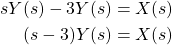which in turns means that the H(s) is: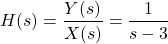There is only one pole at s = 3, and so the corresponding pole-zero plot is: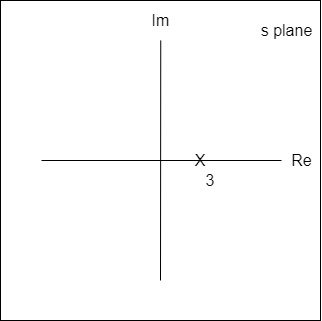### 2.

For a causal system, the ROC must be to-the-right of the right-most pole, whilst for an anti-causal system it must be to-the-left of the left-most pole.

As such, the two choices for ROCs are: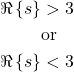Graphically, this leads to the pole-zero plots shown below.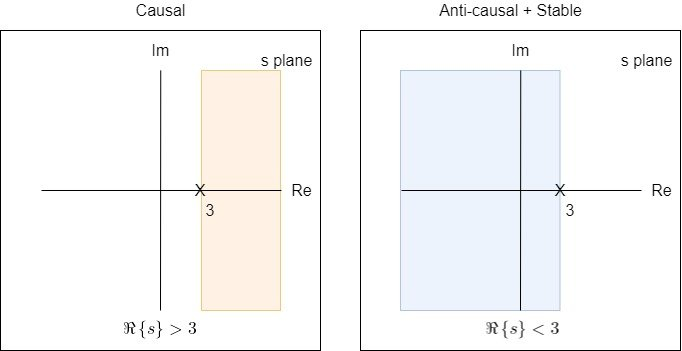The left-sided plane also satisfies stability, as the imaginary (jω) axis is included in the ROC.

### 3.

The function H(s) is one of the known Laplace Transform cases, and so taking the inverse Laplace Transform is as simple as: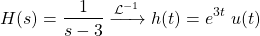## RESOURCES:

### Images

Block diagrams and other visualizations were made using draw.io and GeoGebra

## Previous articles of the series

### Laplace and Z Transforms

• Laplace Transform → Laplace Transform, Region of Convergence (ROC)
• Laplace Transform Properties → Linearity, Time- and Frequency-Shifting, Time-Scaling, Complex Conjugation, Multiplication and Convolution, Differentation in Time- and Frequency-Domain, Integration in Time-Domain, Initial and Final Value Theorems
• LTI System Analysis using Laplace Transform → System Properties (Causality, Stability) and ROC, LCCDE Representation and Laplace Transform, First-Order and Second-Order System Analysis

## Final words | Next up

And this is actually it for today's post!

Next time we will start getting into the Z-Transform, which is a somewhat discrete-time version of the Laplace Transform...

See Ya!Keep on drifting!